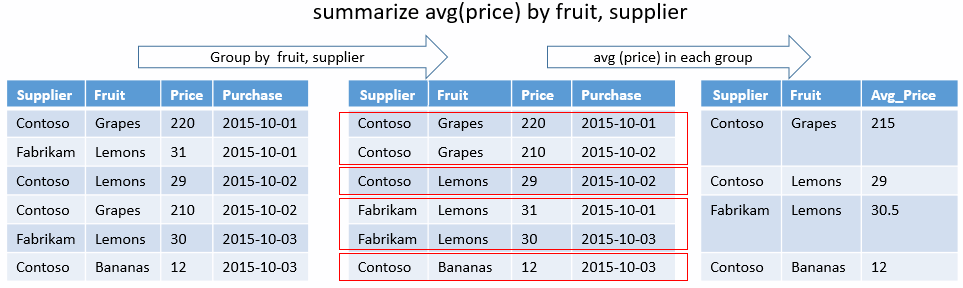# summarize 运算符

``````Sales | summarize NumTransactions=count(), Total=sum(UnitPrice * NumUnits) by Fruit, StartOfMonth=startofmonth(SellDateTime)
``````

``````T | summarize count() by price_range=bin(price, 10.0)
``````

## 语法

T`| summarize` [SummarizeParameters] [[Column`=`] Aggregation [`,` ...]] [`by` [Column`=`] GroupExpression [`,` ...]]

## 参数

• Column：结果列的可选名称。 默认为派生自表达式的名称。

• 聚合：聚合函数（例如 `count()``avg()`）的调用，以列名作为参数。

• GroupExpression：一个可以引用输入数据的标量表达式。 所有组表达式有多少个不同的值，输出就会包含多少个记录。

• SummarizeParameters：零个或更多（以空格分隔）Name`=`Value 形式的参数，用于控制行为。 支持以下参数：

名称 描述
`hint.num_partitions` 指定用于在群集节点上共享查询负载的分区数。 请参阅随机执行查询
`hint.shufflekey=<key>` `shufflekey` 查询使用键将数据分区，在群集节点上共享查询负载。 请参阅 shuffle 查询
`hint.strategy=shuffle` `shuffle` 策略查询会在群集节点上共享查询负载，其中的每个节点将处理一个数据分区。 请参阅 shuffle 查询

• 如果未提供 GroupExpression，则输出将为单个（空）行。
• 如果提供了 GroupExpression，则输出将不包含任何行。

## 返回

• 尽管可为聚合和分组表达式提供任意表达式，但使用简单列名称或将 `bin()` 应用于数值列会更加高效。
• 不再支持自动地每小时对日期/时间列进行分箱。 请改用显式分箱。 例如 `summarize by bin(timestamp, 1h)`

## 对默认值进行聚合

`count()`, `countif()`, `dcount()`, `dcountif()` 0
`make_bag()`, `make_bag_if()`, `make_list()`, `make_list_if()`, `make_set()`, `make_set_if()` 空的动态数组 ([])

## 示例### 唯一组合

``````Activities | summarize by ActivityType, completionStatus
``````

`ActivityType` `completionStatus`
`dancing` `started`
`singing` `started`
`dancing` `abandoned`
`singing` `completed`

### 最小和最大时间戳

``````Activities | summarize Min = min(Timestamp), Max = max(Timestamp)
``````

`Min` `Max`
`1975-06-09 09:21:45` `2015-12-24 23:45:00`

### 非重复计数

``````Activities | summarize cities=dcount(city) by continent
``````

`cities` `continent`
`4290` `Asia`
`3267` `Europe`
`2673` `North America`

### 直方图

``````Activities | summarize count() by ActivityType, length=bin(Duration, 10m)
``````

`count_` `ActivityType` `length`
`354` `dancing` `0:00:00.000`
`23` `singing` `0:00:00.000`
`2717` `dancing` `0:10:00.000`
`341` `singing` `0:10:00.000`
`725` `dancing` `0:20:00.000`
`2876` `singing` `0:20:00.000`
...

### 对默认值进行聚合

`summarize` 运算符的输入至少有一个空的分组依据键时，其结果也将为空。

``````datatable(x:long)[]
| summarize any_x=take_any(x), arg_max_x=arg_max(x, *), arg_min_x=arg_min(x, *), avg(x), buildschema(todynamic(tostring(x))), max(x), min(x), percentile(x, 55), hll(x) ,stdev(x), sum(x), sumif(x, x > 0), tdigest(x), variance(x)
``````

any_x arg_max_x arg_min_x avg_x schema_x max_x min_x percentile_x_55 hll_x stdev_x sum_x sumif_x tdigest_x variance_x
NaN 0 0 0 0

`avg_x(x)` 的结果为 `NaN`，因为被除以 0。

``````datatable(x:long)[]
| summarize  count(x), countif(x > 0) , dcount(x), dcountif(x, x > 0)
``````

count_x countif_ dcount_x dcountif_x
0 0 0 0
``````datatable(x:long)[]
| summarize  make_set(x), make_list(x)
``````

set_x list_x
[] []

``````range x from 1 to 2 step 1
| extend y = iff(x == 1, real(null), real(5))
| summarize sum(y), avg(y)
``````

sum_y avg_y
5 5

``````range x from 1 to 2 step 1
| extend y = iff(x == 1, real(null), real(5))
| summarize count(y)
``````

count_y
2
``````range x from 1 to 2 step 1
| extend y = iff(x == 1, real(null), real(5))
| summarize make_set(y), make_set(y)
``````

set_y set_y1
[5.0] [5.0]